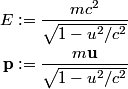## Solution to 1986 Problem 68

 In special relativity, the energy and the momentum of a particle with mass are given by:\begin{align*}E &:= \frac{mc^2}{\sqrt{1 - u^2/c^2}} \\\mathbf{p} &:= \frac{m \mathbf{u}}{\sqrt{1 - u^2/c^2}}\end{alig...So, if u = c, both the energy and the momentum of the particle are infinite, which is absurd. Therefore, answer (A) is correct. The existence of the photon, which can be detected, means that answers (D) and (E) is wrong. There is no reason that the spin of such a particle must equal the spin of a photon. So, answer (B) is wrong. Answer (C) is wrong because the particle, if it is elementary, does not have a surface."